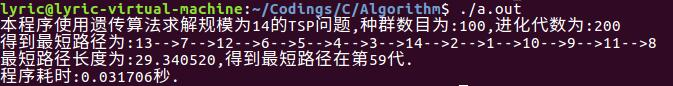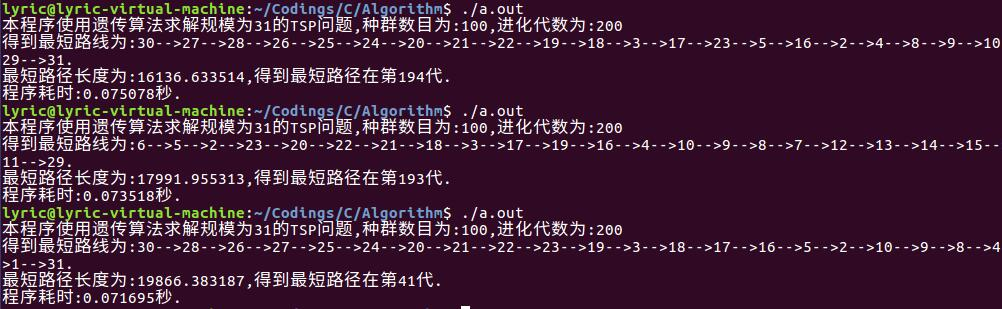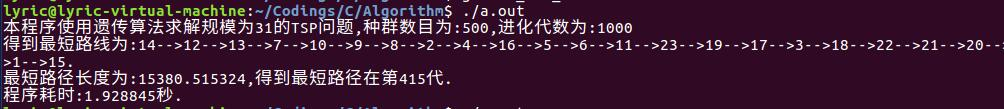# 遗传算法的C语言实现(二)-----以求解TSP问题为例

上一次我们使用遗传算法求解了一个较为复杂的多元非线性函数的极值问题，也基本了解了遗传算法的实现基本步骤。这一次，我再以经典的TSP问题为例，更加深入地说明遗传算法中选择、交叉、变异等核心步骤的实现。而且这一次解决的是离散型问题，上一次解决的是连续型问题，刚好形成对照。

首先介绍一下TSP问题。TSP(traveling salesman problem,旅行商问题)是典型的NP完全问题，即其最坏情况下的时间复杂度随着问题规模的增大按指数方式增长，到目前为止还没有找到一个多项式时间的有效算法。TSP问题可以描述为：已知n个城市之间的相互距离，某一旅行商从某一个城市出发，访问每个城市一次且仅一次，最后回到出发的城市，如何安排才能使其所走的路线最短。换言之，就是寻找一条遍历n个城市的路径，或者说搜索自然子集X={1,2,...,n}(X的元素表示对n个城市的编号)的一个排列P(X)={V1,V2,....,Vn},使得Td=∑d(Vi,Vi+1)+d(Vn,V1)取最小值，其中,d(Vi,Vi+1)表示城市Vi到Vi+1的距离。TSP问题不仅仅是旅行商问题，其他许多NP完全问题也可以归结为TSP问题，如邮路问题，装配线上的螺母问题和产品的生产安排问题等等，也使得TSP问题的求解具有更加广泛的实际意义。

再来说针对TSP问题使用遗传算法的步骤。

（1）编码问题：由于这是一个离散型的问题，我们采用整数编码的方式，用1~n来表示n个城市，1~n的任意一个排列就构成了问题的一个解。可以知道，对于n个城市的TSP问题，一共有n!种不同的路线。

(2)种群初始化：对于N个个体的种群，随机给出N个问题的解（相当于是染色体）作为初始种群。这里具体采用的方法是：1,2，...,n作为第一个个体，然后2,3，..n分别与1交换位置得到n-1个解，从2开始，3,4,...,n分别与2交换位置得到n-2个解，依次类推。（如果这样还不够初始种群的数量，可以再考虑n,n-1,...,1这个序列，然后再按照相同的方法生成，等等）

(3)适应度函数：设一个解遍历初始行走的总距离为D，则适应度fitness=1/D.即总距离越高，适应度越低，总距离越低（解越好），适应度越高。

(4) 选择操作：个体被选中的概率与适应度成正比，适应度越高，个体被选中的概率越大。这里仍然采用轮盘赌法。

(5) 交叉操作：交叉操作是遗传算法最重要的操作，是产生新个体的主要来源，直接关系到算法的全局寻优能力，这里采用部分映射交叉。比如对于n=10的情况，对于两个路径:            1 2 4 5 6 3   9 10 8 7

3 9 7 6 8 10 5  1  2  4

1 9 7 6 6 3   9 10 8 7

3 2 4 5 8 10 5  1  2  4

1 9 7 6 5 3   2 10 8 4

3 2 4 5 8 10 6  1  7

(6)变异操作:变异操作采取对于一个染色体(即个体)随机选取两个基因进行交换的策略。比如对于染色体:

2 4 6 10 3 1 9 7 8 5

2 4 7 10 3 1 9 6 8 5

（7） 进化逆转操作：这个是标准的遗传算法没有的，是我们为了加速进化而加入的一个操作。这里的进化是指逆转操作具有单向性，即只有逆转之后个体变得更优才会执行逆转操作，否则逆转无效。具体的方法是，随机产生[1,10](这里仍然以10个城市为例)之间的两个随机数r1和r2(其实也是允许相同的，只是r1,r2相同之后，逆转自然无效,设置交叉变异都是无效的，但是这不会经常发生),然后将r1和r2之间的基因进行反向排序。比如对于染色体:

1 3 4 2 10  9 8 7 6 5

r1=3,r2=5，它们之间的基因(标红位置)反向排列之后得到的染色体如下:

1 3 10 2 4  9 8 7 6 5

/*
*遗传算法(GA) 解决TSP 问题
*案例参考自《MATLAB 智能算法30个案例分析》
*本例以14个城市为例，14个城市的位置坐标如下(括号内第一个元素为X坐标，第二个为纵坐标)：1:(16.47,96.10)  2:(16.47,94.44)  3:(20.09,92.54)
*4:(22.39,93.37) 5:(25.23,97.24)  6:(22.00,96.05) 7:(20.47,97.02)  8:(17.20,96.29) 9:(16.30,97.38) 10:(14.05,98.12) 11:(16.53,97.38)
*12:(21.52,95.59)  13:(19.41,97.13)  14:(20.09,92.55)
*遗传算法实现的步骤为:(1)编码 (2) 种群初始化 (3) 构造适应度函数 (4) 选择操作 (5) 交叉操作 (6) 变异操作 (7) 进化逆转操作
* 具体实现的步骤这里不详细说，参考《MATLAB 智能算法30个案例分析》P38 - P40
* update in 16/12/4
* author:Lyrichu
* email:919987476@qq.com
*/
#include<stdio.h>
#include<stdlib.h>
#include<math.h>
#include<time.h>
#define maxgen 200  // 最大进化代数
#define sizepop 100 // 种群数目
#define pcross 0.6 // 交叉概率
#define pmutation 0.1 // 变异概率
#define lenchrom 14 // 染色体长度(这里即为城市个数)
double city_pos[lenchrom] = {{16.47,96.10},{16.47,94.44},{20.09,92.54},{22.39,93.37},{25.23,97.24},{22.00,96.05},{20.47,97.02},
{17.20,96.29},{16.30,97.38},{14.05,98.12},{16.53,97.38},{21.52,95.59},{19.41,97.13},{20.09,92.55}}; // 定义二维数组存放14个城市的X、Y坐标
int chrom[sizepop][lenchrom]; // 种群
int best_result[lenchrom]; // 最佳路线
double min_distance; // 最短路径长度

// 函数声明
void init(void); // 种群初始化函数
double distance(double *,double *); // 计算两个城市之间的距离
double * min(double *); // 计算距离数组的最小值
double path_len(int *); // 计算某一个方案的路径长度，适应度函数为路线长度的倒数
void Choice(int [sizepop][lenchrom]); // 选择操作
void Cross(int [sizepop][lenchrom]); // 交叉操作
void Mutation(int [sizepop][lenchrom]); // 变异操作
void Reverse(int [sizepop][lenchrom]); // 逆转操作

// 种群初始化
void init(void)
{
int num = 0;
while(num < sizepop)
{
for(int i=0;i<sizepop;i++)
for(int j=0;j<lenchrom;j++)
chrom[i][j] = j+1;
num++;
for(int i=0;i<lenchrom-1;i++)
{
for(int j=i+1;j<lenchrom;j++)
{
int temp = chrom[num][i];
chrom[num][i] = chrom[num][j];
chrom[num][j] = temp; // 交换第num个个体的第i个元素和第j个元素
num++;
if(num >= sizepop)
break;
}
if(num >= sizepop)
break;
}
// 如果经过上面的循环还是无法产生足够的初始个体，则随机再补充一部分
// 具体方式就是选择两个基因位置，然后交换
while(num < sizepop)
{
double r1 = ((double)rand())/(RAND_MAX+1.0);
double r2 = ((double)rand())/(RAND_MAX+1.0);
int p1 = (int)(lenchrom*r1); // 位置1
int p2 = (int)(lenchrom*r2); // 位置2
int temp = chrom[num][p1];
chrom[num][p1] = chrom[num][p2];
chrom[num][p2] = temp;    // 交换基因位置
num++;
}
}
}

// 距离函数
double distance(double * city1,double * city2)
{
double x1 = *city1;
double y1 = *(city1+1);
double x2 = *(city2);
double y2 = *(city2+1);
double dis = sqrt((x1-x2)*(x1-x2)+(y1-y2)*(y1-y2));
return dis;
}
// min()函数
double * min(double * arr)
{
static double best_index;
double min_dis = *arr;
double min_index = 0;
for(int i=1;i<sizepop;i++)
{
double dis = *(arr+i);
if(dis < min_dis)
{
min_dis = dis;
min_index = i;
}
}
best_index = min_index;
best_index = min_dis;
return best_index;
}

// 计算路径长度
double path_len(int * arr)
{
double path = 0; // 初始化路径长度
int index = *arr; // 定位到第一个数字(城市序号)
for(int i=0;i<lenchrom-1;i++)
{
int index1 = *(arr+i);
int index2 = *(arr+i+1);
double dis = distance(city_pos[index1-1],city_pos[index2-1]);
path += dis;
}
int last_index = *(arr+lenchrom-1); // 最后一个城市序号
int first_index = *arr; // 第一个城市序号
double last_dis = distance(city_pos[last_index-1],city_pos[first_index-1]);
path = path + last_dis;
return path; // 返回总的路径长度
}

// 选择操作
void Choice(int chrom[sizepop][lenchrom])
{
double pick;
double choice_arr[sizepop][lenchrom];
double fit_pro[sizepop];
double sum = 0;
double fit[sizepop]; // 适应度函数数组(距离的倒数)
for(int j=0;j<sizepop;j++)
{
double path = path_len(chrom[j]);
double fitness = 1/path;
fit[j] = fitness;
sum += fitness;
}
for(int j=0;j<sizepop;j++)
{
fit_pro[j] = fit[j]/sum; // 概率数组
}
// 开始轮盘赌
for(int i=0;i<sizepop;i++)
{
pick = ((double)rand())/RAND_MAX; // 0到1之间的随机数
for(int j=0;j<sizepop;j++)
{
pick = pick - fit_pro[j];
if(pick<=0)
{
for(int k=0;k<lenchrom;k++)
choice_arr[i][k] = chrom[j][k]; // 选中一个个体
break;
}
}

}
for(int i=0;i<sizepop;i++)
{
for(int j=0;j<lenchrom;j++)
chrom[i][j] = choice_arr[i][j];
}
}

//交叉操作
void Cross(int chrom[sizepop][lenchrom])
{
double pick;
double pick1,pick2;
int choice1,choice2;
int pos1,pos2;
int temp;
int conflict1[lenchrom]; // 冲突位置
int conflict2[lenchrom];
int num1,num2;
int index1,index2;
int move = 0; // 当前移动的位置
while(move<lenchrom-1)
{
pick = ((double)rand())/RAND_MAX; // 用于决定是否进行交叉操作
if(pick > pcross)
{
move += 2;
continue; // 本次不进行交叉
}
// 采用部分映射杂交
choice1 = move; // 用于选取杂交的两个父代
choice2 = move+1; // 注意避免下标越界
pick1 = ((double)rand())/(RAND_MAX+1.0);
pick2 = ((double)rand())/(RAND_MAX+1.0);
pos1 = (int)(pick1*lenchrom); // 用于确定两个杂交点的位置
pos2 = (int)(pick2*lenchrom);
while(pos1 > lenchrom -2 || pos1 < 1)
{
pick1 = ((double)rand())/(RAND_MAX+1.0);
pos1 = (int)(pick1*lenchrom);
}
while(pos2 > lenchrom -2 || pos2 < 1)
{
pick2 = ((double)rand())/(RAND_MAX+1.0);
pos2 = (int)(pick2*lenchrom);
}
if(pos1 > pos2)
{
temp = pos1;
pos1 = pos2;
pos2 = temp; // 交换pos1和pos2的位置
}
for(int j=pos1;j<=pos2;j++)
{
temp = chrom[choice1][j];
chrom[choice1][j] = chrom[choice2][j];
chrom[choice2][j] = temp; // 逐个交换顺序
}
num1 = 0;
num2 = 0;
if(pos1 > 0 && pos2 < lenchrom-1)
{
for(int j =0;j<=pos1-1;j++)
{
for(int k=pos1;k<=pos2;k++)
{
if(chrom[choice1][j] == chrom[choice1][k])
{
conflict1[num1] = j;
num1++;
}
if(chrom[choice2][j] == chrom[choice2][k])
{
conflict2[num2] = j;
num2++;
}
}
}
for(int j=pos2+1;j<lenchrom;j++)
{
for(int k=pos1;k<=pos2;k++)
{
if(chrom[choice1][j] == chrom[choice1][k])
{
conflict1[num1] = j;
num1++;
}
if(chrom[choice2][j] == chrom[choice2][k])
{
conflict2[num2] = j;
num2++;
}
}

}
}
if((num1 == num2) && num1 > 0)
{
for(int j=0;j<num1;j++)
{
index1 = conflict1[j];
index2 = conflict2[j];
temp = chrom[choice1][index1]; // 交换冲突的位置
chrom[choice1][index1] = chrom[choice2][index2];
chrom[choice2][index2] = temp;
}
}
move += 2;
}
}

// 变异操作
// 变异策略采取随机选取两个点，将其对换位置
void Mutation(int chrom[sizepop][lenchrom])
{
double pick,pick1,pick2;
int pos1,pos2,temp;
for(int i=0;i<sizepop;i++)
{
pick = ((double)rand())/RAND_MAX; // 用于判断是否进行变异操作
if(pick > pmutation)
continue;
pick1 = ((double)rand())/(RAND_MAX+1.0);
pick2 = ((double)rand())/(RAND_MAX+1.0);
pos1 = (int)(pick1*lenchrom); // 选取进行变异的位置
pos2 = (int)(pick2*lenchrom);
while(pos1 > lenchrom-1)
{
pick1 = ((double)rand())/(RAND_MAX+1.0);
pos1 = (int)(pick1*lenchrom);
}
while(pos2 > lenchrom-1)
{
pick2 = ((double)rand())/(RAND_MAX+1.0);
pos2 = (int)(pick2*lenchrom);
}
temp = chrom[i][pos1];
chrom[i][pos1] = chrom[i][pos2];
chrom[i][pos2] = temp;
}
}

// 进化逆转操作
void Reverse(int chrom[sizepop][lenchrom])
{
double pick1,pick2;
double dis,reverse_dis;
int n;
int flag,pos1,pos2,temp;
int reverse_arr[lenchrom];

for(int i=0;i<sizepop;i++)
{
flag = 0; // 用于控制本次逆转是否有效
while(flag == 0)
{
pick1 = ((double)rand())/(RAND_MAX+1.0);
pick2 = ((double)rand())/(RAND_MAX+1.0);
pos1 = (int)(pick1*lenchrom); // 选取进行逆转操作的位置
pos2 = (int)(pick2*lenchrom);
while(pos1 > lenchrom-1)
{
pick1 = ((double)rand())/(RAND_MAX+1.0);
pos1 = (int)(pick1*lenchrom);
}
while(pos2 > lenchrom -1)
{
pick2 = ((double)rand())/(RAND_MAX+1.0);
pos2 = (int)(pick2*lenchrom);
}
if(pos1 > pos2)
{
temp = pos1;
pos1 = pos2;
pos2 = temp; // 交换使得pos1 <= pos2
}
if(pos1 < pos2)
{
for(int j=0;j<lenchrom;j++)
reverse_arr[j] = chrom[i][j]; // 复制数组
n = 0; // 逆转数目
for(int j=pos1;j<=pos2;j++)
{
reverse_arr[j] = chrom[i][pos2-n]; // 逆转数组
n++;
}
reverse_dis = path_len(reverse_arr); // 逆转之后的距离
dis = path_len(chrom[i]); // 原始距离
if(reverse_dis < dis)
{
for(int j=0;j<lenchrom;j++)
chrom[i][j] = reverse_arr[j]; // 更新个体
}
}
flag = 1;
}

}
}

// 主函数
int main(void)
{
time_t start,finish;
start = clock(); // 开始计时
srand((unsigned)time(NULL)); // 初始化随机数种子
init(); // 初始化种群

int best_fit_index = 0; //最短路径出现代数
double distance_arr[sizepop];
double dis;
for(int j=0;j<sizepop;j++)
{
dis = path_len(chrom[j]);
distance_arr[j] = dis;
}
double * best_index = min(distance_arr); // 计算最短路径及序号
min_distance = *(best_index+1); // 最短路径
int index = (int)(*best_index); // 最短路径序号
for(int j=0;j<lenchrom;j++)
best_result[j] = chrom[index][j]; // 最短路径序列

// 开始进化
double * new_arr;
double new_min_dis;
int new_index;
for(int i=0;i<maxgen;i++)
{
Choice(chrom); // 选择
Cross(chrom); //交叉
Mutation(chrom); //变异
Reverse(chrom); // 逆转操作
for(int j=0;j<sizepop;j++)
distance_arr[j] = path_len(chrom[j]); // 距离数组
new_arr = min(distance_arr);
new_min_dis = *(new_arr+1); //新的最短路径
if(new_min_dis < min_distance)
{
min_distance = new_min_dis; // 更新最短路径
new_index =(int)(*new_arr);
for(int j=0;j<lenchrom;j++)
best_result[j] = chrom[new_index][j]; // 更新最短路径序列
best_fit_index = i+1; // 最短路径代数
}
}
finish = clock(); // 计算结束
double duration = ((double)(finish-start))/CLOCKS_PER_SEC; // 计算耗时
printf("本程序使用遗传算法求解规模为%d的TSP问题,种群数目为:%d,进化代数为:%d\n",lenchrom,sizepop,maxgen);
printf("得到最短路径为:%d-->%d-->%d-->%d-->%d-->%d-->%d-->%d-->%d-->%d-->%d-->%d-->%d-->%d\n",best_result,best_result,best_result,
best_result,best_result,best_result,best_result,best_result,best_result,best_result,best_result,best_result,
best_result,best_result);
printf("最短路径长度为:%lf,得到最短路径在第%d代.\n",min_distance,best_fit_index);
printf("程序耗时:%lf秒.\n",duration);
return 0;
}07-071万+

#### 一个简单实用的遗传算法c程序（转载）

05-063109

#### 遗传算法C语言源码

12-151万+

#### 利用动态规划法求解旅行商问题（TSP）的C语言实现（一）

05-051843

#### 利用贪心算法求解TSP问题(C语言实现)©️2020 CSDN 皮肤主题: 大白 设计师: CSDN官方博客点击重新获取扫码支付1.余额是钱包充值的虚拟货币，按照1:1的比例进行支付金额的抵扣。
2.余额无法直接购买下载，可以购买VIP、C币套餐、付费专栏及课程。余额充值